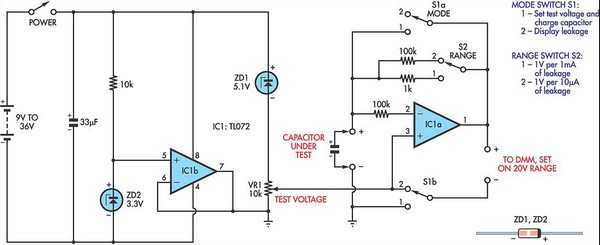# Learning Electronics

Learn to build electronic circuits

# Capacitor Leakage Adaptor For DMMs

Used with a DMM on the 20V range, this circuit gives a rapid and direct measure of the leakage current of capacitors. There are two ranges, with maximum readings of about 20µA and 2mA, and the test voltage can be varied. This lets you test leakage at or near the capacitor's rated voltage. In addition, the circuit can help determine the amount of internal electro-chemical activity, which reduces the capacitor's lifespan. For example, one 0.33F 5.5V super capacitor I tested has an open-circuit voltage that rises exponentially to about 0.8V over a period of 10 days.

Note:

Super capacitors are technically called electro-chemical capacitors but they store energy electrostatically like other capacitors. To quantify the internal electro-chemical activity of a capacitor using this circuit, simply measure the capacitor's "leakage" with the test voltage set to zero. If the reading is negative, the capacitor is self-charging with its plus terminal becoming positive with respect to its minus terminal. If the reading is greater than zero, the capacitor is self-charging with its minus terminal becoming positive with respect to its plus terminal. In the circuit, the 10kO potentiometer (VR1) adjusts the test voltage.

Zener diode ZD1 limits the maximum test voltage to ensure that the output of IC1a can swing to at least 2V above the test voltage. IC1b and associated components derive the ground rail from the single-ended supply. The negative supply voltage is fixed at -3.3V by ZD2 to give more range to the test voltage, which is derived from the positive supply. The circuit will operate from any voltage in the range 9-36V but keep in mind that the maximum test voltage is 8.4V less than the supply voltage. With S1 in position 1, IC1a is configured as a unity gain buffer and the DMM reads its output voltage.

Circuit diagram: